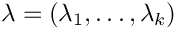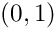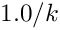#Boost C++ Libraries

...one of the most highly regarded and expertly designed C++ library projects in the world.

This is the documentation for a snapshot of the master branch, built from commit 25d613dfbc.

## Class template hyperexponential_distribution

boost::random::hyperexponential_distribution

## Synopsis

```// In header: <boost/random/hyperexponential_distribution.hpp>

template<typename RealT = double>
class hyperexponential_distribution {
public:
// types
typedef RealT result_type;
typedef RealT input_type;

// member classes/structs/unions

class param_type {
public:
// types
typedef hyperexponential_distribution distribution_type;

// construct/copy/destruct
param_type();
template<typename ProbIterT, typename RateIterT>
param_type(ProbIterT, ProbIterT, RateIterT, RateIterT);
template<typename ProbRangeT, typename RateRangeT>
param_type(ProbRangeT const &, RateRangeT const &,
typename boost::disable_if_c< boost::has_pre_increment< ProbRangeT >::value||boost::has_pre_increment< RateRangeT >::value >::type * = 0);
template<typename RateIterT>
param_type(RateIterT, RateIterT,
typename boost::enable_if_c< boost::has_pre_increment< RateIterT >::value >::type * = 0);
template<typename RateRangeT> param_type(RateRangeT const &);
param_type(std::initializer_list< RealT >, std::initializer_list< RealT >);
param_type(std::initializer_list< RealT >);

// public member functions
std::vector< RealT > probabilities() const;
std::vector< RealT > rates() const;

// friend functions
template<typename CharT, typename Traits>
friend std::basic_ostream< CharT, Traits > &
operator<<(std::basic_ostream< CharT, Traits > &, const param_type &);
template<typename CharT, typename Traits>
friend std::basic_istream< CharT, Traits > &
operator>>(std::basic_istream< CharT, Traits > &, const param_type &);
friend bool operator==(const param_type &, const param_type &);
friend bool operator!=(const param_type &, const param_type &);
};

// construct/copy/destruct
hyperexponential_distribution();
template<typename ProbIterT, typename RateIterT>
hyperexponential_distribution(ProbIterT, ProbIterT, RateIterT, RateIterT);
template<typename ProbRangeT, typename RateRangeT>
hyperexponential_distribution(ProbRangeT const &, RateRangeT const &,
typename boost::disable_if_c< boost::has_pre_increment< ProbRangeT >::value||boost::has_pre_increment< RateRangeT >::value >::type * = 0);
template<typename RateIterT>
hyperexponential_distribution(RateIterT, RateIterT,
typename boost::enable_if_c< boost::has_pre_increment< RateIterT >::value >::type * = 0);
template<typename RateRangeT>
hyperexponential_distribution(RateRangeT const &);
explicit hyperexponential_distribution(param_type const &);
hyperexponential_distribution(std::initializer_list< RealT > const &,
std::initializer_list< RealT > const &);
hyperexponential_distribution(std::initializer_list< RealT > const &);

// public member functions
template<typename URNG> RealT operator()(URNG &) const;
template<typename URNG> RealT operator()(URNG &, const param_type &) const;
std::size_t num_phases() const;
std::vector< RealT > probabilities() const;
std::vector< RealT > rates() const;
RealT min() const;
RealT max() const;
param_type param() const;
void param(param_type const &);
void reset();

// friend functions
template<typename CharT, typename Traits>
friend std::basic_ostream< CharT, Traits > &
operator<<(std::basic_ostream< CharT, Traits > &,
const hyperexponential_distribution &);
template<typename CharT, typename Traits>
friend std::basic_istream< CharT, Traits > &
operator>>(std::basic_istream< CharT, Traits > &,
const hyperexponential_distribution &);
friend bool operator==(const hyperexponential_distribution &,
const hyperexponential_distribution &);
friend bool operator!=(const hyperexponential_distribution &,
const hyperexponential_distribution &);
};```

## Description

The hyperexponential distribution is a real-valued continuous distribution with two parameters, the phase probability vector `probs` and the rate vector `rates`.

A-phase hyperexponential distribution is a mixture ofexponential distributions. For this reason, it is also referred to as mixed exponential distribution or parallel-phase exponential distribution.

A-phase hyperexponential distribution is characterized by two parameters, namely a phase probability vectorand a rate vector.

A-phase hyperexponential distribution is frequently used in queueing theory to model the distribution of the superposition ofindependent events, like, for instance, the service time distribution of a queueing station withservers in parallel where the-th server is chosen with probabilityand its service time distribution is an exponential distribution with rate(Allen,1990; Papadopolous et al.,1993; Trivedi,2002).

For instance, CPUs service-time distribution in a computing system has often been observed to possess such a distribution (Rosin,1965). Also, the arrival of different types of customer to a single queueing station is often modeled as a hyperexponential distribution (Papadopolous et al.,1993). Similarly, if a product manufactured in several parallel assemply lines and the outputs are merged, the failure density of the overall product is likely to be hyperexponential (Trivedi,2002).

Finally, since the hyperexponential distribution exhibits a high Coefficient of Variation (CoV), that is a CoV > 1, it is especially suited to fit empirical data with large CoV (Feitelson,2014; Wolski et al.,2013) and to approximate long-tail probability distributions (Feldmann et al.,1998).

A-phase hyperexponential distribution has a probability density function

Equation 34.1.where:

•is the number of phases and also the size of the input vector parameters,

•is the phase probability vector parameter, and

•is the rate vector parameter.

Given a-phase hyperexponential distribution with phase probability vectorand rate vector, the random variate generation algorithm consists of the following steps (Tyszer,1999):

1. Generate a random variableuniformly distribution on the interval.

2. Useto select the appropriate(e.g., the alias method can possibly be used for this step).

3. Generate an exponentially distributed random variablewith rate parameter.

4. Return.

References:

1. A.O. Allen, Probability, Statistics, and Queuing Theory with Computer Science Applications, Second Edition, Academic Press, 1990.

2. Boost C++ Libraries, Boost.Math / Statistical Distributions: Hyperexponential Distribution, Online: http://www.boost.org/doc/libs/release/libs/math/doc/html/dist.html , 2014.

3. D.G. Feitelson, Workload Modeling for Computer Systems Performance Evaluation, Cambridge University Press, 2014

4. A. Feldmann and W. Whitt, Fitting mixtures of exponentials to long-tail distributions to analyze network performance models, Performance Evaluation 31(3-4):245, doi:10.1016/S0166-5316(97)00003-5, 1998.

5. H.T. Papadopolous, C. Heavey and J. Browne, Queueing Theory in Manufacturing Systems Analysis and Design, Chapman & Hall/CRC, 1993, p. 35.

6. R.F. Rosin, Determining a computing center environment, Communications of the ACM 8(7):463-468, 1965.

7. K.S. Trivedi, Probability and Statistics with Reliability, Queueing, and Computer Science Applications, John Wiley & Sons, Inc., 2002.

8. J. Tyszer, Object-Oriented Computer Simulation of Discrete-Event Systems, Springer, 1999.

9. Wikipedia, Hyperexponential Distribution, Online: http://en.wikipedia.org/wiki/Hyperexponential_distribution , 2014.

10. Wolfram Mathematica, Hyperexponential Distribution, Online: http://reference.wolfram.com/language/ref/HyperexponentialDistribution.html , 2014.

Marco Guazzone (marco.guazzone@gmail.com)

### `hyperexponential_distribution` public construct/copy/destruct

1. `hyperexponential_distribution();`

Constructs a 1-phase `hyperexponential_distribution` (i.e., an exponential distribution) with rate 1.

2. ```template<typename ProbIterT, typename RateIterT>
hyperexponential_distribution(ProbIterT prob_first, ProbIterT prob_last,
RateIterT rate_first, RateIterT rate_last);```

Constructs a `hyperexponential_distribution` from the phase probability vector and rate vector parameters of the distribution.

The phase probability vector parameter is given by the range defined by [prob_first, prob_last) iterator pair, and the rate vector parameter is given by the range defined by [rate_first, rate_last) iterator pair.

References:

1. ISO, ISO/IEC 14882-2014: Information technology - Programming languages - C++, 2014

Parameters:

 `prob_first` The iterator to the beginning of the range of non-negative real elements representing the phase probabilities; if elements don't sum to 1, they are normalized. `prob_last` The iterator to the ending of the range of non-negative real elements representing the phase probabilities; if elements don't sum to 1, they are normalized. `rate_first` The iterator to the beginning of the range of non-negative real elements representing the rates. `rate_last` The iterator to the ending of the range of non-negative real elements representing the rates.

Template Parameters:

 `ProbIterT` Must meet the requirements of `InputIterator` concept (ISO,2014,sec. 24.2.3 [input.iterators]). `RateIterT` Must meet the requirements of `InputIterator` concept (ISO,2014,sec. 24.2.3 [input.iterators]).
3. ```template<typename ProbRangeT, typename RateRangeT>
hyperexponential_distribution(ProbRangeT const & prob_range,
RateRangeT const & rate_range,
typename boost::disable_if_c< boost::has_pre_increment< ProbRangeT >::value||boost::has_pre_increment< RateRangeT >::value >::type * = 0);```

Constructs a `hyperexponential_distribution` from the phase probability vector and rate vector parameters of the distribution.

The phase probability vector parameter is given by the range defined by prob_range, and the rate vector parameter is given by the range defined by rate_range.

NoteThe final `disable_if` parameter is an implementation detail that differentiates between this two argument constructor and the iterator-based two argument constructor described below.

Parameters:

 `prob_range` The range of non-negative real elements representing the phase probabilities; if elements don't sum to 1, they are normalized. `rate_range` The range of positive real elements representing the rates.

Template Parameters:

 `ProbRangeT` Must meet the requirements of Range concept. `RateRangeT` Must meet the requirements of Range concept.
4. ```template<typename RateIterT>
hyperexponential_distribution(RateIterT rate_first, RateIterT rate_last,
typename boost::enable_if_c< boost::has_pre_increment< RateIterT >::value >::type * = 0);```

Constructs a `hyperexponential_distribution` from the rate vector parameter of the distribution and with equal phase probabilities.

The rate vector parameter is given by the range defined by [rate_first, rate_last) iterator pair, and the phase probability vector parameter is set to the equal phase probabilities (i.e., to a vector of the same lengthof the rate vector and with each element set to).

NoteThe final `disable_if` parameter is an implementation detail that differentiates between this two argument constructor and the range-based two argument constructor described above.

References:

1. ISO, ISO/IEC 14882-2014: Information technology - Programming languages - C++, 2014

Parameters:

 `rate_first` The iterator to the beginning of the range of non-negative real elements representing the rates. `rate_last` The iterator to the ending of the range of non-negative real elements representing the rates.

Template Parameters:

 `RateIterT` Must meet the requirements of `InputIterator` concept (ISO,2014,sec. 24.2.3 [input.iterators]).
5. ```template<typename RateRangeT>
hyperexponential_distribution(RateRangeT const & rate_range);```

Constructs a `param_type` from the "rates" parameters of the distribution and with equal phase probabilities.

The rate vector parameter is given by the range defined by rate_range, and the phase probability vector parameter is set to the equal phase probabilities (i.e., to a vector of the same lengthof the rate vector and with each element set to).

Parameters:

 `rate_range` The range of positive real elements representing the rates.

Template Parameters:

 `RateRangeT` Must meet the requirements of Range concept.
6. `explicit hyperexponential_distribution(param_type const & param);`

Constructs a `hyperexponential_distribution` from its parameters.

Parameters:

 `param` The parameters of the distribution.
7. ```hyperexponential_distribution(std::initializer_list< RealT > const & l1,
std::initializer_list< RealT > const & l2);```

Constructs a `hyperexponential_distribution` from the phase probability vector and rate vector parameters of the distribution.

The phase probability vector parameter is given by the brace-init-list (ISO,2014,sec. 8.5.4 [dcl.init.list]) defined by l1, and the rate vector parameter is given by the brace-init-list (ISO,2014,sec. 8.5.4 [dcl.init.list]) defined by l2.

References:

1. ISO, ISO/IEC 14882-2014: Information technology - Programming languages - C++, 2014

Parameters:

 `l1` The initializer list for inizializing the phase probability vector. `l2` The initializer list for inizializing the rate vector.
8. `hyperexponential_distribution(std::initializer_list< RealT > const & l1);`

Constructs a `hyperexponential_distribution` from the rate vector parameter of the distribution and with equal phase probabilities.

The rate vector parameter is given by the brace-init-list (ISO,2014,sec. 8.5.4 [dcl.init.list]) defined by l1, and the phase probability vector parameter is set to the equal phase probabilities (i.e., to a vector of the same lengthof the rate vector and with each element set to).

References:

1. ISO, ISO/IEC 14882-2014: Information technology - Programming languages - C++, 2014

Parameters:

 `l1` The initializer list for inizializing the rate vector.

### `hyperexponential_distribution` public member functions

1. `template<typename URNG> RealT operator()(URNG & urng) const;`

Gets a random variate distributed according to the hyperexponential distribution.

Parameters:

 `urng` A uniform random number generator object.

Template Parameters:

 `URNG` Must meet the requirements of uniform random number generator .

Returns:

A random variate distributed according to the hyperexponential distribution.

2. ```template<typename URNG>
RealT operator()(URNG & urng, const param_type & param) const;```

Gets a random variate distributed according to the hyperexponential distribution with parameters specified by `param`.

Parameters:

 `param` A distribution parameter object. `urng` A uniform random number generator object.

Template Parameters:

 `URNG` Must meet the requirements of uniform random number generator .

Returns:

A random variate distributed according to the hyperexponential distribution. distribution with parameters specified by `param`.

3. `std::size_t num_phases() const;`

Returns the number of phases of the distribution.

4. `std::vector< RealT > probabilities() const;`

Returns the phase probability vector parameter of the distribution.

5. `std::vector< RealT > rates() const;`

Returns the rate vector parameter of the distribution.

6. `RealT min() const;`

Returns the smallest value that the distribution can produce.

7. `RealT max() const;`

Returns the largest value that the distribution can produce.

8. `param_type param() const;`

Returns the parameters of the distribution.

9. `void param(param_type const & param);`

Sets the parameters of the distribution.

10. `void reset();`

Effects: Subsequent uses of the distribution do not depend on values produced by any engine prior to invoking reset.

### `hyperexponential_distribution` friend functions

1. ```template<typename CharT, typename Traits>
friend std::basic_ostream< CharT, Traits > &
operator<<(std::basic_ostream< CharT, Traits > & os,
const hyperexponential_distribution & hd);```

Writes an `hyperexponential_distribution` to a `std::ostream`.

2. ```template<typename CharT, typename Traits>
friend std::basic_istream< CharT, Traits > &
operator>>(std::basic_istream< CharT, Traits > & is,
const hyperexponential_distribution & hd);```

Reads an `hyperexponential_distribution` from a `std::istream`.

3. ```friend bool operator==(const hyperexponential_distribution & lhs,
const hyperexponential_distribution & rhs);```

Returns true if the two instances of `hyperexponential_distribution` will return identical sequences of values given equal generators.

4. ```friend bool operator!=(const hyperexponential_distribution & lhs,
const hyperexponential_distribution & rhs);```

Returns true if the two instances of `hyperexponential_distribution` will return different sequences of values given equal generators.